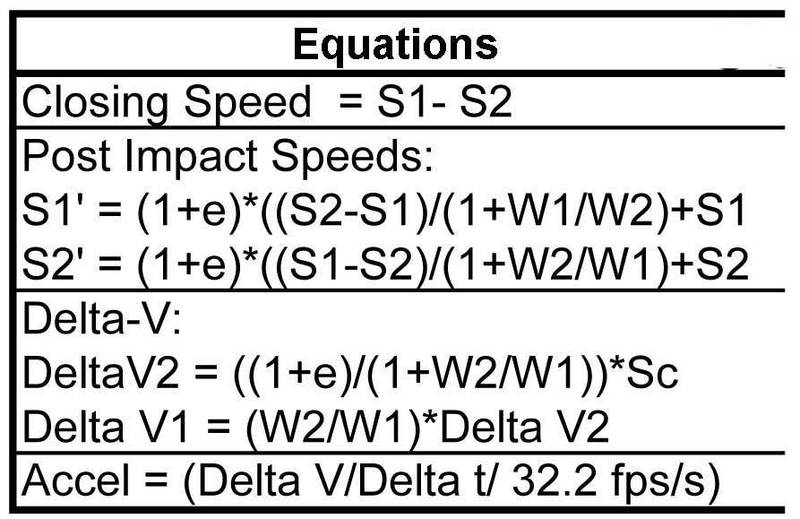# Newbie here with a question

• I
• Steve Max

#### Steve Max

Can someone help me clear up where this formula originates and if it is correct?
Has to do with a low speed in line bullet vehicle front end impacting the rear of a stopped target vehicle.
S1' (post impact) = (1 + e) X ((S2 - S1) / (1 + W1 / W2)) + S1
S = Speed in mph
W = Weight of vehicles 1 (bullet) and 2 (target)
e = restitution (a decimal value from 0 to 1)

Welcome to PF;
The equation originates from wherever you got it from... I sort-of know what you mean, but in order to answer your question properly, we need to know where you saw it.

It is likely that the equation comes from conservation of momentum and a bunch of experiments in which cars were crashed into each other.

The equation tells me that vehicle 2 hits vehicle 1 from behind, and bounces off.
If #1 is "stopped" then put S1=0. For cars, "e" is something you'd have to estimate (it is determined empirically.)

•berkeman
Thank you for the help. You are indeed on the right track.
The formula was offered by a biomechanical engineer and I don't fully understand it.
I believe its origin is from a momentum-energy-restitution approach to solving for post impact speeds of vehicles involved in a rear end impact.
Veh 1 is the bullet at speed. Veh 2 is the stopped (no brakes) target. Veh 1 front strikes veh 2 rear.
In this scenario, veh 1 (4760 lbs) is at 10 mph when its front strikes the rear of a stopped veh 2 (4967 lbs).
I'm trying to understand the methodology and know if it is appropriate.I think you need to be specific - I do not understand what you want to calculate.
Try just using your normal language instead of attempting to use the jargon.

Initially it sounded like "a vehicle" as in an automobile colliding with another one as both travel along the road ... OR, a moving automobile colliding with a stationary one... NOW it sounds like a stationary one has been shot.
What you are hoping to use the information for is also important for what we tell you.

I'll give you a couple of examples of how these equations get derived:

1. A bullet m speed u collides with a stationary vehicle M and is embedded in it.
##mu = (M+m)v## ... this is conservation of momentum. Conservation of energy is usually not useful in macroscopic collisions.
The speed of the two after the collision is: ##v=mu/(M+m) = u/(1+(M/m))##

2. A bullet m speed u collides with a stationary vehicle M of coefficient of restitution e, bounces directly back ...

##mu = Mv-mu'## ... by definition: ##e=(v+u')/u## so ##u' = eu - v## so
The speed of the vehicle after the collision is: ##v=mu(1+e)/(M+m) = (1+e)u/(1+(M/m))## ... the speed of the bullet would have m/M instead of M/m.

I appreciate and value your help, but I am a little confused with your symbol use.
can S1 be the pre-impact speed of veh1 (Bullet). S1 = 10mph
can S2 be the pre-impact speed of veh2 (Target). S2 = 0mph
S1' = post impact speed veh1
S2' = post impact speed veh2
Looking for S1' and S2' first off.

Fair enough: I'll do this in two parts ...
The equation you have been given has object 1 and object 2 are the target and the bullet respectively, with primed symbols representing the post-impact quantities. Using that W2'=W2 tells you that the post-impact weight of the bullet is the same as the pre-impact weight.
Then: S1=0, S1'=v, S2=u, S2'=u', W1=Mg, W2=mg.
... where I write two symbols together as in "mg" I imply a multiplication - in this case "mass times gravity".
This is the standard way to do physics.

So for example 1: the bullet strikes a stationary target and sticks to it:
conservation of momentum: (W2/g)×S2 = (W1/g)S1' + (W2/g)S2'. After some algebra this means: S1' = W2×S2/(W1+W2)
I would write that as $$v=\frac{mu}{m+M}=\frac{u}{1+\frac{M}{m}}$$ ... see how that looks tidier?

Last edited:
I appreciate and value your help, but I am a little confused with your symbol use.
can S1 be the pre-impact speed of veh1 (Bullet). S1 = 10mph
can S2 be the pre-impact speed of veh2 (Target). S2 = 0mph
So this would be backwards from the equation you were given.
So: "no", you cannot use the equations like that.

Using (from example 2 above) $$v = \frac{mu(1+e)}{m+M}$$
... then v is the post-collision speed of the target: one of the things you want to find.
u=10mph
You need to know m (mass of bullet), M (mass of target), and e (coefficient of restitution), as well.
Veh 1 is the bullet at speed. Veh 2 is the stopped (no brakes) target. Veh 1 front strikes veh 2 rear.
In this scenario, veh 1 (4760 lbs) is at 10 mph when its front strikes the rear of a stopped veh 2 (4967 lbs).
So m=4760lbs and M=4967lbs ... still need ##e##.

So there is not enough information to complete the calculation.

Again: what do you want to know these things for?